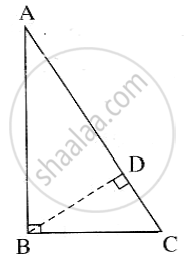# Prove That, in a Right-angled Triangle, the Square of Hypotenuse is Equal to the Sum of the Square of Remaining Two Sides - Geometry

Prove that, in a right-angled triangle, the square of the hypotenuse is equal to the sum of the square of remaining two sides

#### SolutionDraw perpendicular BD from the vertex B to the side AC. A - D

In right-angled triangle ABC

seg BD ⊥ hypotenuse AC.

∴ by the similarity in right-angled triangles

triangle ABC ~ triangle ADB ~ triangle BDC

Now, triangle ABC ~ triangle ADB

:. (AB)/(AD) = (AC)/(AB)     ......(c.s.s.t)

∴ AB2 = AC x AD   ......(1)

Also, triangleABC ~ triangleBDC

:. (BC)/(DC) = (AC)/(BC)     ......(c.s.s.t)

:. BC^2 = AC xx DC     ......(2)

From (1) and (2),

AB^2 + BC^2 = AC xx AD + AC xx DC

= AC xx (AD + DC)

= AC xx AC        ......(A-D-C)

:. AB^2 + BC^2 = AC^2

i.e,  AC^2 = AB^2 + BC^2

Concept: Right-angled Triangles and Pythagoras Property
Is there an error in this question or solution?